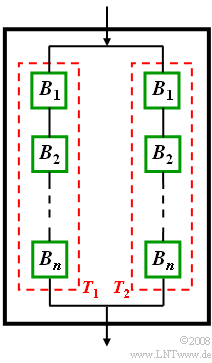# Exercise 1.5Z: Probabilities of DefaultFunctional circuit diagram of a device with two identical parts  $T_1$  and  $T_2$

A sub-device is composed of the components   $B_1, \ B_2,\ \text{...} \ , B_n$  where the respective functionality can be assumed to be independent of all other components.

• Assume that all components default with equal probability  $p_{\rm B}$.
• Sub-device  $T_1$  functions only if all  $n$  components are functional.

To increase reliability,  important assemblies are often duplicated.  The overall device  $G$  can be described in terms of set theory as follows:

$$G = T_1 \cup T_2.$$

This means:   The overall device  $G$  is already operational if at least one of the two identical sub-devices  $(T_1$  or  $T_2)$  is functional.

Hints:

• The topic of this chapter is illustrated with examples in the  (German language)  learning video
Statistische Abhängigkeit und Unabhängigkeit   $\Rightarrow$   "Statistical dependence and independence".
• Explanations:
1. Component  (German:  "Bauteil"   ⇒   subscript "B")
2. Sub-device  (German:  "Teilgerät"   ⇒   subscript "T")
3. Overall device (German: "Gesamtgerät"   ⇒   subscript "G")

### Questions

1

The default probability  $p_{\rm G}$  of the overall device must not be greater than  $0.04\%$.
How large may then the default probabilities  $p_{\rm T}$  of the two identical sub-devices existing in parallel be at most?

 $p_\text{T, max} \ = \$ $\ \%$

2

Let the default probability of all components be  $\underline{p_{\rm B} = 0.1}$.  Let each sub-device consist of  $n = 3$  components.
Calculate the probability  $p_{\rm T}$  exactly that a sub-device defaults.

 $p_{\rm T} \ = \$ $\ \%$

3

What value is obtained for  $\underline{p_{\rm B} = 0.01}$?  In what form can you approximate  $p_{\rm T}$  for small values of  $p_{\rm B}$ ?

 $p_{\rm T} \ = \$ $\ \%$

4

Now apply  $p_{\rm B} = 0.4\%$  for the default probability of all components.   What is the maximum number of components the sub-device can contain?  $p_{\rm T} ≤ 2\%$  is to hold.

 $n \ = \$

### Solution

#### Solution

(1)  Since the two sub-devices default independently,  set-theoretically holds:

$${\rm Pr(}G \text{ drops out)} = {\rm Pr(}T_1 \text{ drops out)} \cdot {\rm Pr(}T_2 \text{ drops out)}.$$
• Moreover,  since the sub-devices  $T_1$  and  $T_2$  are identical in construction,  they default with the same probability  $p_{\rm T}.$  It follows that:
$$p_{\rm G} = \it p_{\rm T}^{\rm 2} \hspace{0.5cm} \Rightarrow \hspace{0.5cm} \rm \it p_{\rm T,\hspace{0.1cm}max}= \sqrt{\it p_{\rm G}} \le \rm\sqrt{0.0004} \hspace{0.15cm}\underline {= 2\%}.$$

(2)  This result is easier to determine using the complementary event:

$$\rm Pr(\it T_{\rm 1}\hspace{0.1cm}\rm functions) = \rm Pr(\it B_{\rm 1} \hspace{0.1cm}\rm functions \cap \it B_{\rm 2} \hspace{0.1cm} \rm functions \cap \it B_{\rm 3}\hspace{0.1cm} \rm functions).$$
$$\Rightarrow 1- p_{\rm T}= (1-p_{\rm B})^{3} \hspace{0.3cm} \Rightarrow \hspace{0.3cm} 1-p_{\rm T}=(0.9)^3= 0.729 \hspace{0.3cm} \Rightarrow \hspace{0.3cm} p_{\rm T}\hspace{0.15cm}\underline {= 0.271 = 27.1\%}.$$

(3)  With  $p_{\rm B} = 0.01$,  we obtain  $p_{\rm T}\hspace{0.15cm}\underline {= 2.97\%}.$

• In general,  the approximation is:  $p_{\rm T} \approx n \cdot p_{\rm B}\; (= 3\%)$.

(4)  With the approximation of the last subtask   ⇒   $\underline{n = 5}$  follows directly.

• For larger  $p_{\rm B}$,  one would have to proceed as follows:
$$0.996^{\it n}\ge 0.98 \hspace{0.5cm} \rm\Rightarrow \hspace{0.5cm} \it n\le\rm\frac{log(0.98)}{log(0.996)} = 5.0406\hspace{0.15cm}\underline { \approx 5}.$$